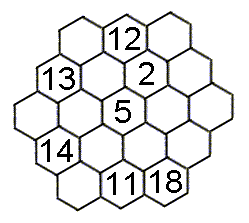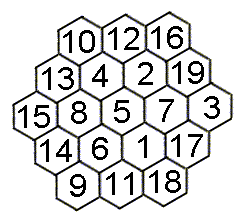### PRIZE PUZZLE FOR SEPTEMBER 2005

#### THE MAGIC HEXAGONStrange as it may seem, the 19 hexagons at the left can be filled with the numbers 1 to 19 to make each of the rows and diagonals sum to 38, even though there are 3, 4 or 5 hexagons in each row or diagonal.

This puzzle caught my eye in a puzzle book the other day which interested me in several ways. First, I was surprised that it was possible, but it is. Secondly, I wondered what the method would be to solve the diagram from scratch without any help. I don't know, other than using a computer program. Finally, I wondered if other sizes of magic hexagons exist.

The number 38, by the way, as the sum for each row and diagonal, can be computed from the fact that the sum of the numbers from 1 to 19 is 19 x (19+1)/2 = 190 and this must be the total for 5 rows (or diagonals) which makes 38 for each. If we now try to work out the row-sum for other sizes of regular hexagon, except for the trivial case of one single hexagon, all other sizes work out to have a fractional part in the sum. So I conclude that this size of 19 hexagons is unique, which is worth remarking upon (but not proven as I only searched up to a size of 10,981 hexagons with 121 rows using a spreadsheet).

So the puzzle this month is to fill in the missing numbers in the diagram. Scroll down to see the solution.I received 5 correct answers. They were from John Stafford, John Bownas, Gwynn White, Colin Rowe and Rosemary West. The latter two did not wish to participate in the draw so only there were only three for the draw which was won by Gwynn White.

[home] [back]